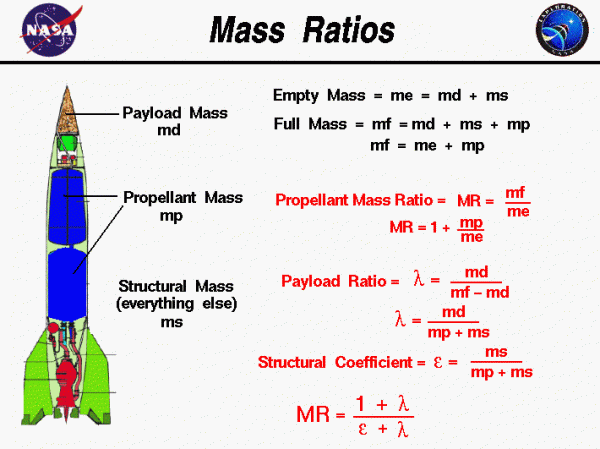# Mass Ratios## Weight

Weight is the force generated by the gravitational attraction of a planet on the mass of a rocket. It is related to the mass through the weight equation and each part of a rocket has a unique weight and mass. For some problems it is important to know the distribution of weight. But for rocket trajectory and stability, we only need to be concerned with the total weight and the location of the center of gravity.

For model rockets and compressed air rockets the weight remains fairly constant during the flight and we can easily compute the weight of the rocket. But for water rockets and full scale rockets the weight changes during launch. When determining the performance of a full-scale rocket, we must account for the change in weight during an engine firing.

## Mass

On the web page which describes the major systems of a rocket, we group the various parts according to function. The major systems include the structural systems, the payload systems, the guidance systems, and the propulsion systems. On this page we group the parts in a slightly different manner according to mass. We assign a mass variable to three major parts; the mass of the payload is noted by md, the total mass of the propellants is noted by mp, and the mass of all of the rest of the rocket, excluding the payload and the propellant, is noted by the structural mass ms.

The engine pumps and nozzle are grouped with the propulsion system according to function but kept with the structure according to mass.

## Changes in Mass of Rocket

We are making the distinction according to mass because the mass of some parts of the rocket are always the same and some change with time. During the launch the propellants are burned and exhausted out the nozzle. To evaluate the performance of a rocket during a burn, we must account for the large change in weight in the equations of motion. Engineers have developed several dimensionless parameters to characterize the weight of a full-scale rocket. We have listed some of these mass ratios on this page.

## Equations of Mass

The empty mass, denoted by me, is the sum of the payload and structural mass of the rocket:

$$\LARGE m_{e}=m_{s}+m_{d}$$

The empty mass is the mass of the vehicle at the end of a burn, assuming all the propellant has been consumed. The full mass, denoted by mf, is the mass at the beginning of the burn and is equal to the sum of the mass of the payload, propellant, and structure:

$$\LARGE m_{f}=m_{s}+m_{d}+m_{p}$$

$$\LARGE m_{f}=m_{e}+m_{p}$$

The propellant mass ratio is denoted MR and is equal to the ratio of the full mass to the empty mass:

$$\LARGE M_{R}=\frac{m_{f}}{m_{e}}$$

$$\LARGE M_{R}=1+\frac{m_{p}}{m_{e}}$$

The ideal rocket equation indicates that the total change in velocity during a burn depends on the natural log of the mass ratio. So, we want the ratio to be a large number to produce a large change in velocity. Another way to look at this parameter is that a large propellant mass ratio implies that the empty weight to hold the propellant is very small.

The payload ratio is denoted by the Greek letter lambda ($$\bf \lambda$$) and is equal to the mass of the payload divided by mass of the propellant and the structure:

$$\LARGE \lambda=\frac{m_{d}}{m_{p}+m_{s}}$$

$$\LARGE \lambda=\frac{m_{d}}{m_{f}-m_{d}}$$

We want the payload ratio to be a large number. This indicates that a large payload can be lofted with a small amount of propellant. The last mass ratio is the structural coefficient, denoted by the Greek letter epsilon ($$\bf \epsilon$$), and equal to the mass of the structure divided by the mass of the structure plus propellant.

$$\LARGE \epsilon=\frac{m_{s}}{m_{p}+m_{s}}$$

This parameter is independent of the payload that is launches and is a measure of the efficiency of the booster design. A small value of this coefficient indicates a good design. With a little algebra, you can show that:

$$\LARGE M_{R}=\frac{1+\lambda}{\epsilon+\lambda}$$

Again, we desire a large payload ratio, a small structural coefficient and a large mass ratio.

Provide feedback